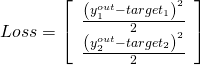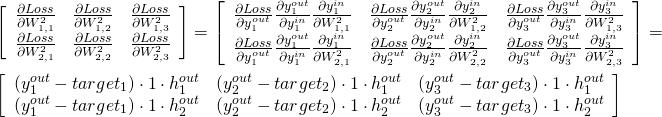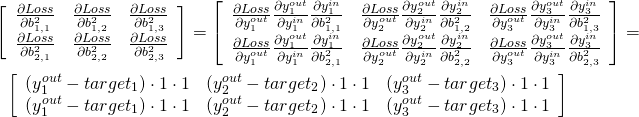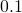# Machine Learning Part 8 — Backpropagation in neural net in SQL

This is the eighth part of the ML series. For your convenience you can find other parts in the table of contents in Part 1 – Linear regression in MXNet

Last time we saw forward propagation in neural net. Today we are going to extend the process to backpropagate the errors. Let’s begin.

We need to add some more definitions to calculate output:

Before we see some SQL code, let’s do some math. We had three layers (input, hidden, output), in input and output layers we used linear activation function. Hidden layer used ReLU.Now let’s calculate partial derivatives to update weights between hidden layer and output layer:Now, the same for biases:That was easy. Now we use learning rate equal toand we can update both weights and biases between hidden layer and output layer.

Similar things go for other updates. If you are lost, you can find great explanation here.

Let’s now see the code:

It is very similar to the solution from previous post. This time in phase 5 we calculate error, in phase 6 we update weights and biases. You can find results here.